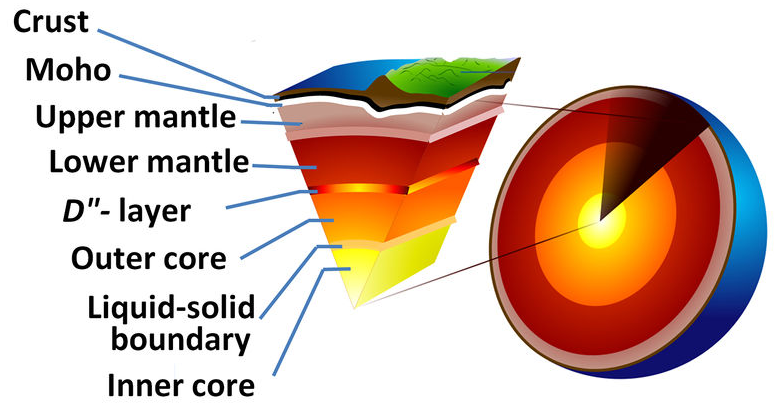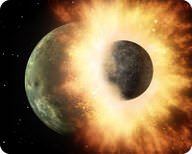Scientists use models to help them understand and explain ideas. Models explain objects or systems in a more simple way. Models often only show only a part of a system. The real situation is more complicated. Models help scientists to make predictions about complex systems. Some models are something that you can see or touch. Other types of models use an idea or numbers. Each type is useful in certain ways.

Scientists create models with computers. Computers can handle enormous amounts of data. This can more accurately represent the real situation. For example, Earth’s climate depends on an enormous number of factors. Climate models can predict how climate will change as certain gases are added to the atmosphere. To test how good a model is, scientists might start a test run at a time in the past. If the model can predict the present it is probably a good model. It is more likely to be accurate when predicting the future.

## Physical Models

A physical model is a representation of something using objects. It can be three-dimensional, like a globe. It can also be a two-dimensional drawing or diagram. Models are usually smaller and simpler than the real object. They most likely leave out some parts, but contain the important parts. In a good model the parts are made or drawn to scale. Physical models allow us to see, feel and move their parts. This allows us to better understand the real system.

An example of a physical model is a drawing of the layers of Earth (figure 1). A drawing helps us to understand the structure of the planet. Yet there are many differences between a drawing and the real thing. The size of a model is much smaller, for example. A drawing also doesn’t give good idea of how substances move. Arrows showing the direction the material moves can help. A physical model is very useful but it can’t explain the real Earth perfectly.Figure 1. Earth’s Center.

## Ideas as ModelsFigure 2. A collision showing a meteor striking Earth.

Some models are based on an idea that helps scientists explain something. A good idea explains all the known facts. An example is how Earth got its Moon. A Mars-sized planet hit Earth and rocky material broke off of both bodies (figure 2). This material orbited Earth and then came together to form the Moon. This is a model of something that happened billions of years ago. It brings together many facts known from our studies of the Moon’s surface. It accounts for the chemical makeup of rocks from the Moon, Earth, and meteorites. The physical properties of Earth and Moon figure in as well. Not all known data fits this model, but much does. There is also more information that we simply don’t yet know.

## Models that Use Numbers

Models may use formulas or equations to describe something. Sometimes math may be the only way to describe it. For example, equations help scientists to explain what happened in the early days of the universe. The universe formed so long ago that math is the only way to describe it. A climate model includes lots of numbers, including temperature readings, ice density, snowfall levels, and humidity. These numbers are put into equations to make a model. The results are used to predict future climate. For example, if there are more clouds, does global temperature go up or down? Models are not perfect because they are simple versions of the real situation. Even so, these models are very useful to scientists. These days, models of complex things are made on computers.

## Contribute!

Did you have an idea for improving this content? We’d love your input.